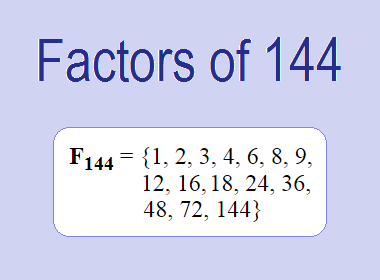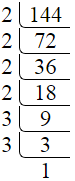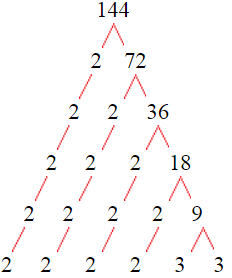# Factors of 144The factors of 144 are 1, 2, 3, 4, 6, 8, 9, 12, 16, 18, 24, 36, 48, 72, and 144 i.e. F144 = {1, 2, 3, 4, 6, 8, 9, 12, 16, 18, 24, 36, 48, 72, 144}. The factors of 144 are all the numbers that can divide 144 without leaving a remainder.

We can check if these numbers are factors of 144 by dividing 144 by each of them. If the result is a whole number, then the number is a factor of 144. Let's do this for each of the numbers listed above:

·        1 is a factor of 144 because 144 divided by 1 is 144.

·        2 is a factor of 144 because 144 divided by 2 is 72.

·        3 is a factor of 144 because 144 divided by 3 is 48.

·        4 is a factor of 144 because 144 divided by 4 is 36.

·        6 is a factor of 144 because 144 divided by 6 is 24.

·        8 is a factor of 144 because 144 divided by 8 is 18.

·        9 is a factor of 144 because 144 divided by 9 is 16.

·        12 is a factor of 144 because 144 divided by 12 is 12.

·        16 is a factor of 144 because 144 divided by 16 is 9.

·        18 is a factor of 144 because 144 divided by 18 is 8.

·        24 is a factor of 144 because 144 divided by 24 is 6.

·        36 is a factor of 144 because 144 divided by 36 is 4.

·        48 is a factor of 144 because 144 divided by 48 is 3.

·        72 is a factor of 144 because 144 divided by 72 is 2.

·        144 is a factor of 144 because 144 divided by 144 is 1.

## How to Find Factors of 144?

1 and the number itself are the factors of every number. So, 1 and 144 are two factors of 144. To find the other factors of 144, we can start by dividing 144 by the numbers between 1 and 144. If we divide 144 by 2, we get a remainder of 0. Therefore, 2 is a factor of 144. If we divide 144 by 3, we get a remainder of 0. Therefore, 3 is also a factor of 144.

Next, we can check if 4 is a factor of 144. If we divide 144 by 4, we get a remainder of 0. Therefore, 4 is also a factor of 144. If we divide 144 by 5, we get a remainder of 4. Therefore, 5 is not a factor of 144. We can continue this process for all the possible factors of 144.

Through this process, we can find that the factors of 144 are 1, 2, 3, 4, 6, 8, 9, 12, 16, 18, 24, 36, 48, 72, and 144. These are the only numbers that can divide 144 without leaving a remainder.

********************

********************

## Properties of the Factors of 144

The factors of 144 have some interesting properties. One of the properties is that the sum of the factors of 144 is equal to 403. We can see this by adding all the factors of 144 together:

1 + 2 + 3 + 4 + 6 + 8 + 9 + 12 + 16 + 18 + 24 + 36 + 48 + 72 + 144 = 403

Another property of the factors of 144 is that the prime factors of 144 are 2, and 3 only and there are all composite numbers except 1, 2, and 3.

## Applications of the Factors of 144

The factors of 144 have several applications in mathematics. One of the applications is in finding the highest common factor (HCF) of two or more numbers. The HCF is the largest factor that two or more numbers have in common. For example, to find the HCF of 144 and 120, we need to find the factors of both numbers and identify the largest factor they have in common. The factors of 144 are 1, 2, 3, 4, 6, 8, 9, 12, 16, 18, 24, 36, 48, 72, and 144. The factors of 120 are 1, 2, 3, 5, 6, 8, 10, 12, 15, 20, 24, 30, 40, 60, and 120. The largest factor that they have in common is 14. Therefore, the HCF of 144 and 120 is 14.

Another application of the factors of 144 is in prime factorization. Prime factorization is the process of expressing a number as the product of its prime factors. The prime factors of 144 are 2, and 3, since these are the only prime numbers that can divide 144 without leaving a remainder. Therefore, we can express 144 as:

144 = 2 × 2 × 2 × 2 × 3 × 3

We can do prime factorization by division and factor tree method also. Here is the prime factorization of 144 by division method,144 = 2 × 2 × 2 × 2 × 3 × 3

Here is the prime factorization of 144 by the factor tree method,144 = 2 × 2 × 2 × 2 × 3 × 3## Conclusion

The factors of 144 are the numbers that can divide 144 without leaving a remainder. The factors of 144 are 1, 2, 3, 4, 6, 8, 9, 12, 16, 18, 24, 36, 48, 72, and 144. The factors of 144 have some interesting properties, such as having a sum of 403. The factors of 144 have several applications in mathematics, such as finding the highest common factor and prime factorization.